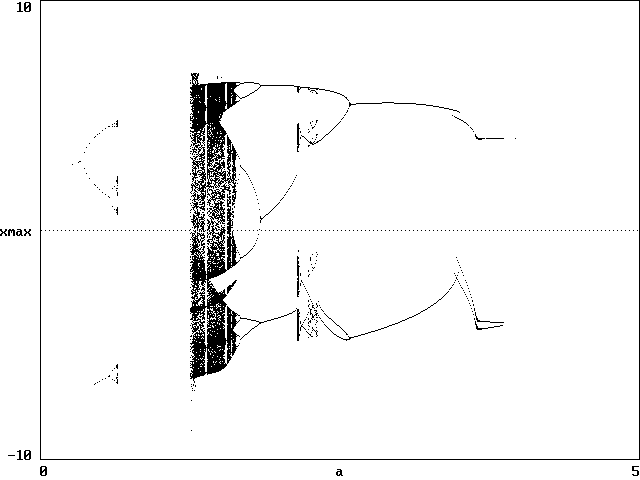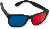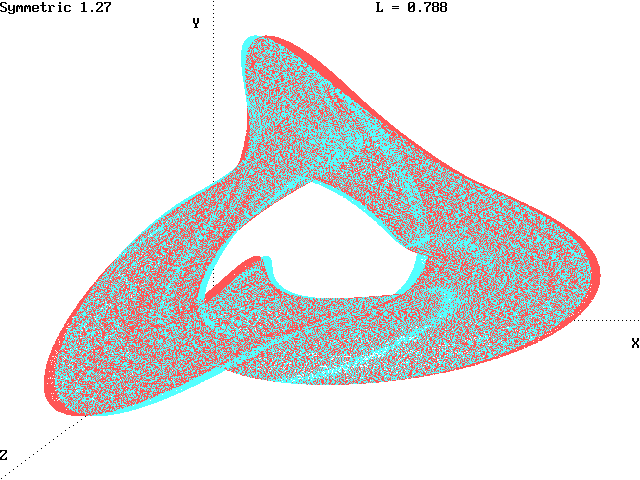# A Symmetric Chaotic Flow

J. C. Sprott
Department of Physics, University of Wisconsin, Madison, WI 53706, USA
August 12, 1997
(Revised July 9, 2004)

On the sci.fractals newsgroup, Arne Dehli Halvorsen proposed a 3-D system of chaotic flows that are symmetric with respect to cyclic interchanges of x, y, and z.  A large number of such systems were examined, and their symmetric attractors are available as 3-D anaglyphs.

One example of such a system is

dx/dt = - ax - 4y - 4z - y2
dy/dt = - ay - 4z - 4x - z2
dz/dt = - az - 4x - 4y - x2

A bifurcation diagram of the above system, in which successive maximum values of x are plotted versus a, was calculated using the BASIC code SYMMETRY.BAS and compiled for DOS as SYMMETRY.EXE.  The result is shown below:The diagram shows a period-doubling route to chaos as a is decreased, with a fully chaotic region at about a = 1.27.  Eight different initial conditions chosen randomly over a cube with a sides of length 10 centered on the origin were used for each value of a.  There appear to be multiple basins of attraction for some values of a.  Below is an anaglyphic view of the attractor for a = 1.27 (use red/blue glasses):Recently, Rene Thomas in Int. J. Bifurcation and Chaos 9, 1889-1905 (1999) proposed an even simpler class of system that possesses the same symmetry:

dx/dt = - ax + f(y)
dy/dt = - ay + f(z)
dz/dt = - az + f(x)

where f is one of a variety of nonlinear functions.  The conservative limit of this sytstem with a = 0 also has chaotic solutions.  A particularly interesting and elegant case is the one with f(x) = sin(x), which has an infinite number of fixed points arranged on a 3-D lattice.  The chaotic trajectory percolates within this lattice of unstable steady states.  He calls this "Labyrinth Chaos".

Both of these systems, and many more, are described in J. C. Sprott, Chaos and Time-Series Analysis, Oxford University Press (2003) and in J. C. Sprott, Elegant Chaos, World Scientific (2010).

Back to Sprott's Technical Notes